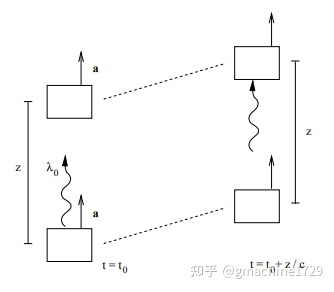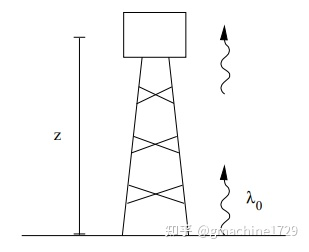## March 28th, 2021

### An intuitive, visual explanation of gravitational redshift

Originally published at 狗和留美者不得入内. You can comment here or there.

I was enticed to learn a bit general relativity after more awareness of the likes of the LIGO detector and gravitational waves, as well as of how astrophysics and cosmology has advanced much more than high energy or particle physics over the past four decades. Moreover, I learned about what appears to be Soviet pre-eminence in astrophysics and cosmology, fields wherein the likes of Sakharov, Zeldovich, Starobinsky, and Linde made seminal contributions. In fact, it seems like the Big Bang Theory is considerably more Soviet than Anglo-American despite the hype of Stephen Hawking and that idiotic TV series with Sheldon Cooper as protagonist.

I never would have considered general relativity (GR) to be worth the time investment, much because I am by the standard of a guy into theoretical physics rather practical and pragmatic actually. But this might actually be a good reason why I would choose general relativity over high energy physics. After all, the LIGO detector as well as detectors of cosmic microwave background radiation which were realized in the 60s are significantly cheaper than the post-80s particle accelerators.

As for deciding what textbook to use, I first downloaded Landau-Lifshitz classical theory of fields. It did not cover very directly or concretely the gravitional redshift and more or less dove straight into the formalism that is heavy on tensors and Riemannian geometry. I wanted to begin by first learning the most concrete ideas behind GR before diving into the mathematics. So in addition to some scattered sources on the internet, I thought of Gerard ‘t Hooft and his page on how to become a good theoretical physicist. From his lecture notes , I learned about Rindler coordinates which describe accelerating frames of reference. The way it was presented was more formal and in terms of infinitesimal changes of the Lorentz transform over infinitesimal changes of time. I was able to more or less follow it with a bit of thinking.

Then I looked at Sean M. Carroll’s lecture notes , wherein it was explained more visually with diagrams of accelerating boxes, with reference to “Doppler shift”. After reading the Wikipedia page on relativistic Doppler shift, gravitational redshift made much sense. It was from that that I grasped the idea behind inertial references frames local to an infinitesimal volume of spacetime. Before I had realized that taking an inertial reference frame that matches the instantaneous speed of an object at some point of time to model it was a common theme in relativity. It is in some sense obvious that over a, in that frame, the object’s displacement of, being second-order, is often negligible.

Understanding of gravitational redshift, or equivalently by Einstein equivalence principle, enlargement of wavelength of light emitted in an accelerating frame in the direction of acceleration requires a correct or appropriate modeling of wavelength. A plane wave has a wavefront, which is some extreme point of the wave in space that is attained one per the period of the wave at the same point on the axis of propagation of the wave. We consider an accelerating boxes as below.As for the bottom box’s emitting a photon of wavelengthwe regard it as emitting a wavefront atand then the next wavefront at. As for the detector on the upper box, it detects the wavelength by measuring with its clock the difference in time of arrival of the two wavefronts and multipling it by the speed of light.

We choose the inertial reference frameat point of emission the first wavefront such that the lower box is instantaneously at rest. We note thatis necessarily extremely small. The wavelengths of radio waves, the electromagnetic waves with the highest wavelengths, range fromto. The speed of light is about. Even the highest wavelength radio wave has a period of only about. The displacement in that case if the accelerating boxes simulate earth’s gravity would be as small as, which we can assume to be negligible compared to the distance between the boxes. Thus we simply assume the next wavefront to be emitted from the same point in frame.

In frame, the initial wavefront reaches the top box at the timesuch that. Ifas we assume,is more or less an infinitesimal change and we again ignore the second order term, which gives. By then, the upper box has accumulated a velocity ofand thus the next wavefront beingbehind it takesamount of time to reach it, according to the clock of frame. Due to time dilation though, the clock at the upper box would measure it asAssuming, we neglect thewhich is a second order term of, which gives the wavelength redshift factor as, which is linear in the distance between the boxes (or the height of the elevator simulating gravity). One can interpret this of course also as the redshift rate with respect to distance.As illustrated from the above diagram, a photon of a given wavelength emitted from upward the ground will also be detected to be redshifted on a sufficiently high tower. This was demonstrated by the Pound-Rebka experiment in 1960.

Recall that by the equivalence principle, a person standing on the ground feels the same as a person standing on a box in free space accelerating atrelative to a true inertial reference frame. Note how the ground on earth is not actually an inertial reference frame due to gravity though it can be regarded as one in the two dimensions orthogonal to the line between the ground the certain of the earth. In contrast, a skydiver in free fall would be in an inertial reference frame. He is, if you neglect the effects of air resistance, accelerating attowards the center of the earth and if he throws a ball upwards during his sky dive, he will view it as moving upwards with no change in velocity, as Newton’s first law would predict.

If one has difficulty visualizing the diagram with the tower, one can an extremely deep pit dug in the vicinity of tower and a person jumping into it at the time of emission of the photon in which case the ground and the top of the tower as both accelerating upwards with magnitude. That person in free fall would be in an inertial frame of reference analogous toin the case of the accelerating boxes.

We conclude with a few notes.

• Simulation of gravity of a planet per an elevator of constant acceleration is only valid if the distance traversed radially along the planet is negligible as far as the change in gravity is concerned.
• Clocks tick faster at places where the strength of gravity is weaker. In the case of an accelerating elevatorin one direction, one can place another elevatoraccelerating in the same direction with a different (positive) magnitude that begins with the same speed relative to an inertial reference frame. Ifaccelerates faster, then its speed after an infinitesimal time change is higher, and thus the factor of time dilation ofrelative to that of the clock at the top ofwould be higher than the factor of time dilation ofrelative to that of the clock at the top of, sincehas attained a higher velocity. Thus the clock corresponding to stronger gravity ticks slower. A clock ticking on Mount Everest or on an airplane would tick faster than a clock on the ground. This phenomenon is called gravitational time dilation.
• We deduced in this article gravitational redshift. Gravitational blueshift, which corresponds to a decrease in a wavelength upon emission of a photon from a tower to the ground, can be deduced analogously.

References